# NCERT Solutions class-11 Chemistry Redox Reactions Part-2## myCBSEguide App

Complete Guide for CBSE Students

NCERT Solutions, NCERT Exemplars, Revison Notes, Free Videos, CBSE Papers, MCQ Tests & more.

16. Why does the following reaction occur?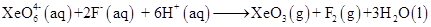What conclusion about the compound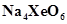(of which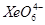is a part) can be drawn from the reaction.

17. Consider the reactions:

(a)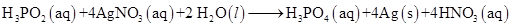(b)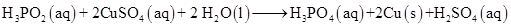(c)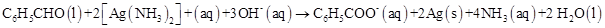(d)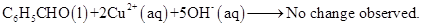What inference do you draw about the behaviour of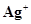and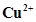from these reactions?

18. Balance the following redox reactions by ion-electron method:

(a)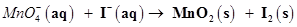(in basic medium)

(b)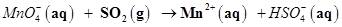(in acidic solution)

(c)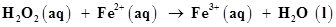(in acidic solution)

(d)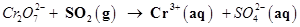(in acidic solution)

19. Balance the following equations in basic medium by ion-electron method and oxidation number methods and identify the oxidising agent and the reducing agent.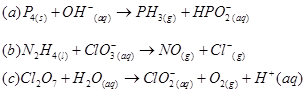20. What sorts of informations can you draw from the following reaction?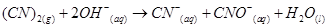21. The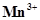ion is unstable in solution and undergoes disproportionation to give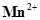,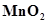, and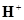ion. Write a balanced ionic equation for the reaction.

22. Consider the elements:

Cs, Ne, I and F

(a) Identify the element that exhibits only negative oxidation state.

(b) Identify the element that exhibits only postive oxidation state.

(c) Identify the element that exhibits both positive and negative oxidation states.

(d) Identify the element which exhibits neither the negative nor does the positive oxidation state.

23. Chlorine is used to purify drinking water. Excess of chlorine is harmful. The excess of chlorine is removed by treating with sulphur dioxide. Present a balanced equation for this redox change taking place in water.

24. Refer to the periodic table given in your book and now answer the following questions:

(a) Select the possible non metals that can show disproportionation reaction.

(b) Select three metals that can show disproportionation reaction.

25. In Ostwald’s process for the manufacture of nitric acid, the first step involves the oxidation of ammonia gas by oxygen gas to give nitric oxide gas and steam. What is the maximum weight of nitric oxide that can be obtained starting only with 10.00 g. of ammonia and 20.00 g of oxygen?

26. Using the standard electrode potentials given in the Table 8.1, predict if the reaction between the following is feasible:

(a)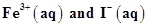(b)(aq) and Cu(s)

(c)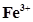(aq) and Cu(s)

(d) Ag(s) and(aq)

(e)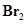(aq) and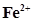(aq)

27. Predict the products of electrolysis in each of the following:

(i) An aqueous solution of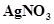with silver electrodes

(ii) An aqueous solutionwith platinum electrodes

(iii) A dilute solution of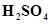with platinum electrodes

(iv) An aqueous solution ofwith platinum electrodes.

28. Arrange the following metals in the order in which they displace each other from the solution of their salts.

Al, Cu, Fe, Mg and Zn.

29. Given the standard electrode potentials,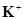/K = -2.93V,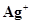/Ag = 0.80V,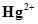/Hg = 0.79V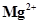/Mg = -2.37V.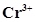/Cr = -0.74V

Arrange these metals in their increasing order of reducing power.

30. Depict the galvanic cell in which the reaction Zn(s) + 2Ag+(aq) → Zn2+(aq) + 2Ag(s) takes place, further show:

(i) which of the electrode is negatively charged,

(ii) the carriers of the current in the cell, and

(iii) individual reaction at each electrode.## Test Generator

Create Papers with your Name & Logo